FreeBuf.COM网络安全行业门户，每日发布专业的安全资讯、技术剖析。FreeBuf+小程序

【JS 逆向百例】猿人学系列 web 比赛第五题：js 混淆 - 乱码增强，详细剖析
2023-03-09 18:16:20## 逆向目标

• 猿人学 - 反混淆刷题平台 Web 第五题：js 混淆，乱码增强

• 目标：抓取全部 5 页直播间热度，计算前 5 名直播间热度的加和

• 主页：https://match.yuanrenxue.com/match/5

• 接口：https://match.yuanrenxue.com/api/match/5?m=XXX&f=XXX

• 逆向参数：

• url 请求参数：m、f

## 逆向过程

### 抓包分析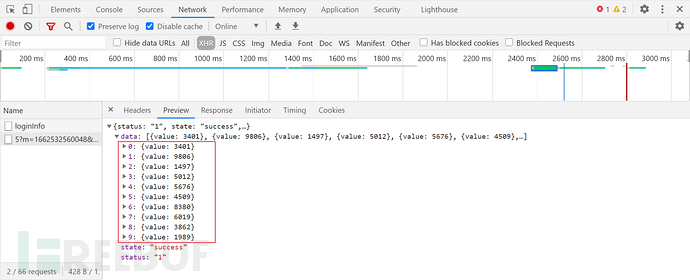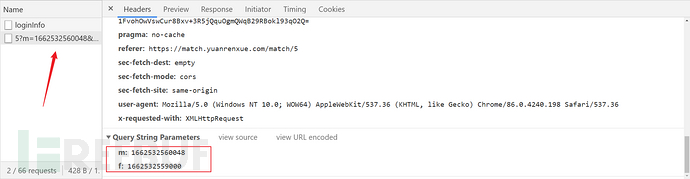## 逆向分析

``````(function () {
'use strict';
set: function (val) {
if (val.indexOf('RM4hZBv0dDon443M') != -1) {
debugger;
}
return val;
},
get: function () {
},
});
})();
``````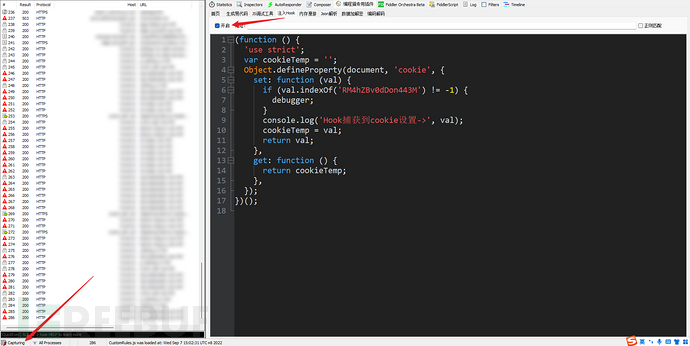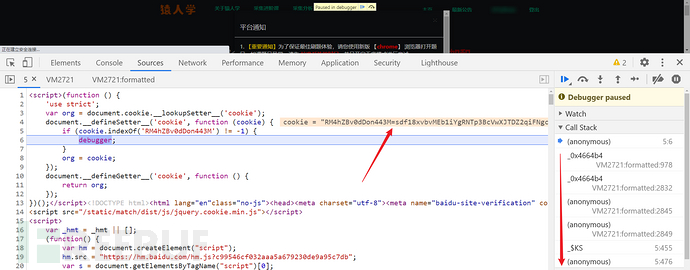``````_0x3d0f3f[_\$Fe] = 'R' + 'M' + '4' + 'h' + 'Z' + 'B' + 'v' + '0' + 'd' + 'D' + 'o' + 'n' + '4' + '4' + '3' + 'M=' + _0x4e96b4['_\$ss'] + ';\x20path=/';
``````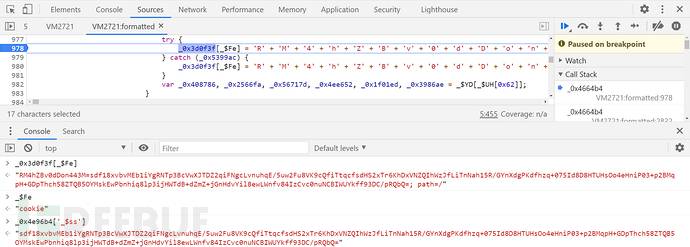• _ 0x4e96b4['_\$ss']：RM4hZBv0dDon443M 参数加密后的值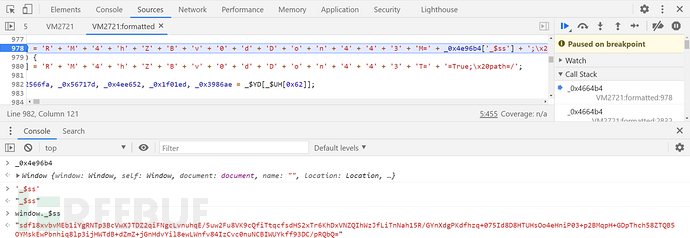``````(function () {
'use strict'
Object.defineProperty(window, '_\$ss', {
set: function (val) {
console.log('Hook捕获到_\$ss的值->', val);
debugger;
},
});
})();
````````````_0x4e96b4['_\$' + _\$UH[0x348][0x1] + _\$UH[0x353][0x1]] = _0x29dd83[_\$UH[0x1f]]();
``````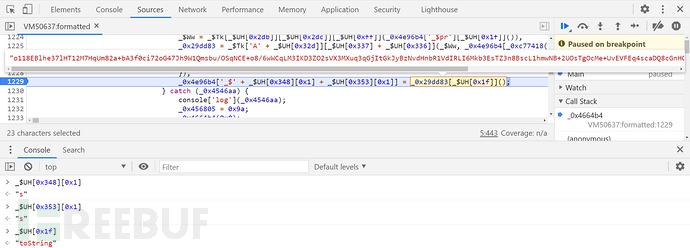• `'_\$s'``_\$UH[0x348][0x1]``_\$UH[0x353][0x1]`组合起来：'_\$ss'

• `_\$UH[0x1f]()`：toString()

• `_0x29dd83[ _\$UH[0x1f]]()`：将 _0x29dd83 生成的值转换为字符串

``````_\$Ww = _\$Tk['enc']['utf-8']['parse'](_0x4e96b4['_\$pr']['toString']()),
_0x29dd83 = _\$Tk['AES'](_\$Ww, _0x4e96b4['_\$qF'], {
'mode': _\$Tk['mode']['ECB'],
}),
_0x4e96b4['_\$ss'] = _0x29dd83['toString']();
``````

• CBC：Cipher Block Chaining（密码块链接模式），是一种循环模式，前一个分组的密文和当前分组的明文异或操作后再加密，这样做的目的是增强破解难度

• PKCS7：在填充时首先获取需要填充的字节长度 = 块长度 - （数据长度 % 块长度）, 在填充字节序列中所有字节填充为需要填充的字节长度值

`_\$Ww`的值由 `_0x4e96b4['_\$pr']`转换为字符串后经过 utf-8 编码得到，其与 key 值 `_0x4e96b4['_\$qF']`都是数组，需要知道这两个数组是怎么生成的，先 ctrl + f 搜索 `_0x4e96b4['_\$qF']`，定义在第 1444 行，内容如下：

``````_0x4e96b4['_\$qF'] = CryptoJS['enc']['Utf8'][_\$UH[0xff]](_0x4e96b4['btoa'](_0x4e96b4['_\$is'])['slice'](0x0, 0x10));
``````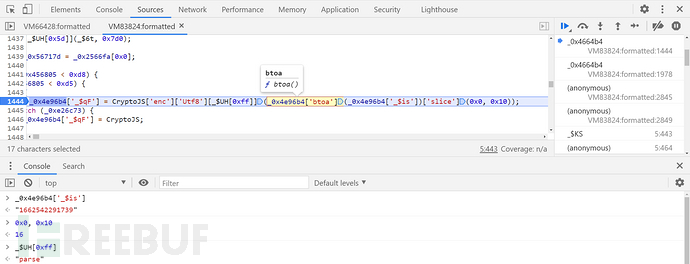``````_0x3d0f3f[_\$Fe] = 'm=' + _0x474032(_\$yw) + ';\x20path=/';
``````

``````_\$yw = _0x2d5f5b()[_\$UH[0x1f]]();
``````

`_\$UH[0x1f]`为 “toString”，`_\$yw`的值是将 `_0x2d5f5b()`函数的返回值转换成了字符串得到的，跟进到该函数定义的位置，搜索后发现在第 279 行，控制台打印后发现这里就是时间戳，所以 `_\$yw`即时间戳：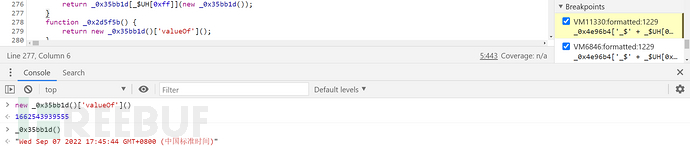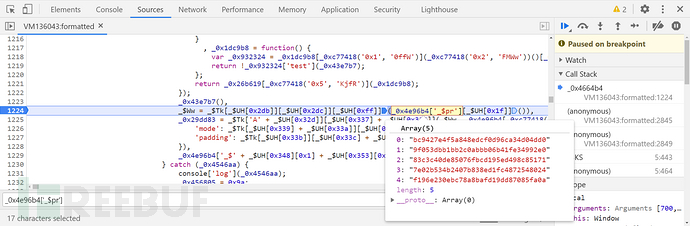``````_0x4e96b4['_\$pr'] = new _0x4d2d2c();
``````

`_0x4d2d2c`在第 224 行定义为 Array，所以这里是创建了一个数组 `_0x4e96b4['_\$pr']`，接着往后找传值的地方，继续运行断点调试，第 1717 行的断点运行了四次传入了四个值：

``````_0x4e96b4['_\$pr']['push'](_0x474032(_\$Wa));
``````

``````Date['parse'](new Date());
``````

``````_0x3d0f3f[_\$Fe] = 'm=' + _0x474032(_\$yw) + ';\x20path=/';
_0x4e96b4['_\$is'] = _\$yw;
_0x4e96b4['_\$pr']['push'](_0x474032(_\$yw));
``````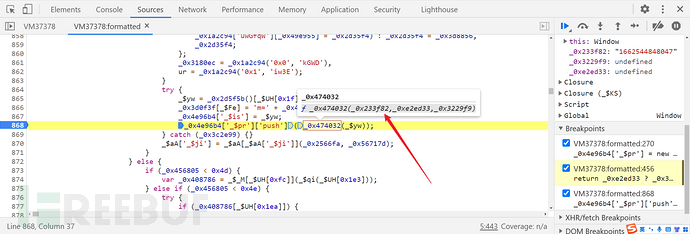``````function _0x474032(_0x233f82, _0xe2ed33, _0x3229f9) {
return _0xe2ed33 ? _0x3229f9 ? v(_0xe2ed33, _0x233f82) : y(_0xe2ed33, _0x233f82) : _0x3229f9 ? _0x41873d(_0x233f82) : _0x37614a(_0x233f82);
}
``````

``````function _0x474032(_0x233f82, _0xe2ed33, _0x3229f9) {
return _0x37614a(_0x233f82);
}
``````

``````function _0x37614a(_0x32e7c1) {
return _0x499969(_0x41873d(_0x32e7c1));
}
``````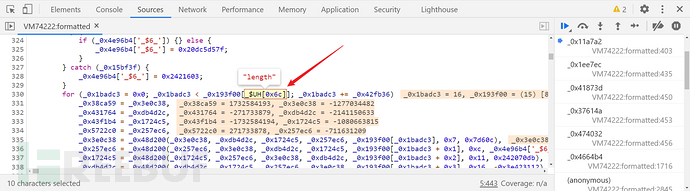``````_\$UH[0x6c] ---> "length"
``````

``````_\$UH = {
8: 'prototype',
15: 'charCodeAt',
31: 'toString',
108: 'length'
}
``````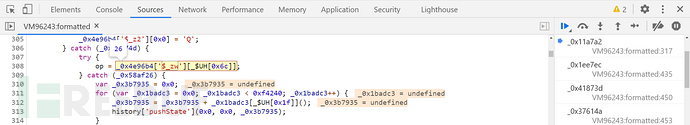op 的值为 26，这里直接将其定义成固定值即可，即 var op = 26;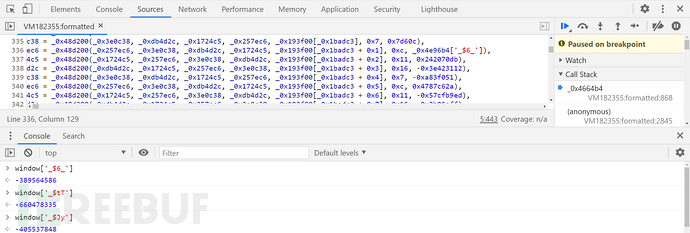``````window['_\$6_'] = -389564586;
window['_\$tT'] = -660478335;
window['_\$Jy'] = -405537848;
``````

``````// 以下函数部分内容过长，此处省略
// 完整代码关注 GitHub：https://github.com/kgepachong/crawler

var CryptoJS = require('crypto-js');

function rm4Encrypt(_\$yw, pr){
var value = Buffer.from(_\$yw).toString('base64').slice(0, 16);
var srcs = CryptoJS.enc.Utf8.parse(pr);
var key = CryptoJS.enc.Utf8.parse(value);
var encrypted = CryptoJS.AES.encrypt(srcs, key, {
mode: CryptoJS.mode.ECB,
});
return encrypted.toString();
}

var _\$yw = new Date().valueOf().toString();
var _\$Wa = Date.parse(new Date())
function pr(){
pr = [];
for (i = 1; i < 5; i++) {
// _\$Wa 传入四个值
pr.push(_0x474032(_\$Wa))
}
// _\$yw 传入一个值
pr.push(_0x474032(_\$yw));
return pr.toString();
}

var RM4hZBv0dDon443M = rm4Encrypt(_\$yw, pr());
// m 为数组传入的最后一个值
var m = pr;
console.log('RM4hZBv0dDon443M 参数加密后的值为: ' + RM4hZBv0dDon443M)
console.log('m 参数的值为: ' + m)
``````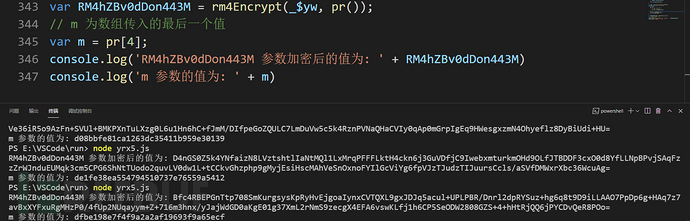### 请求头参数分析

Cookie 中的参数分析完了，还有两个请求参数 m 和 f 没有解决，直接从接口处跟栈，从 Initiator 中跟到 request 里：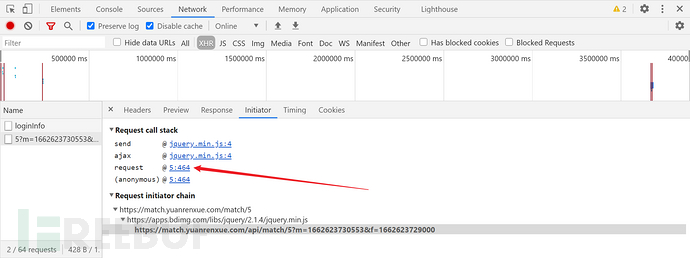``````"m": window._\$is,
"f": window.\$_zw
``````

m 的值是 `window._\$is`，有没感觉似曾相识，就是上文所说的 `_0x4e96b4['_\$is']``_0x4e96b4`就是 window，所以这里 m 的值其实就是 `_\$yw`；f 的值是 `window.\$_zw`，现在需要知道 `\$_zw`的值怎么生成的，局部搜索 `\$_zw`会发现该数组定义在第 611 行，接着往后找，看看数组中的第 23 个是什么，先控制台打印一下内容：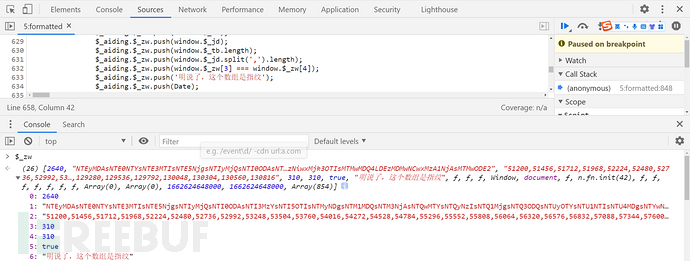``````\$_aiding.\$_zw.push(\$_t1);
``````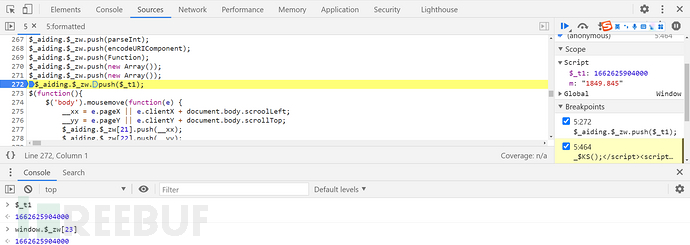``````let \$_t1 = Date.parse(new Date());
``````
• Date.parse(new Date())：获取的时间戳是把毫秒改成 000 显示，如 1662691102000

• new Date().valueOf()：获取了当前包括毫秒的时间戳，如 1662691114310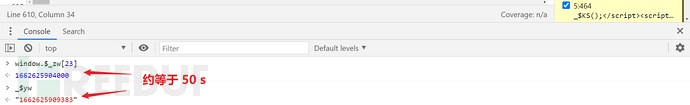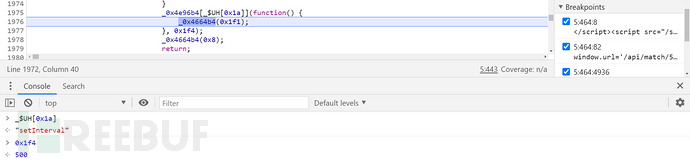## 完整代码

bilibili 关注 K 哥爬虫，小助理手把手视频教学：https://space.bilibili.com/1622879192

GitHub 关注 K 哥爬虫，持续分享爬虫相关代码！欢迎 star ！https://github.com/kgepachong/

### JavaScript 代码

``````var _0x4e96b4 = window = {};
var _0x1171c8 = 0x67452301;
var _0x4dae05 = -0x10325477;
var _0x183a1d = -0x67452302;
var _0xcfa373 = 0x10325476;
var _0x30bc70 = String;

// 以下函数部分内容过长，此处省略
// 完整代码关注 GitHub：https://github.com/kgepachong/crawler

var CryptoJS = require('crypto-js');

function rm4Encrypt(_\$yw, pr){
var value = Buffer.from(_\$yw).toString('base64').slice(0, 16);
var _\$Ww = CryptoJS.enc.Utf8.parse(pr);
var key = CryptoJS.enc.Utf8.parse(value);
var encrypted = CryptoJS.AES.encrypt(_\$Ww, key, {
mode: CryptoJS.mode.ECB,
});
return encrypted.toString();
}

function getParamers() {
pr = [];
for (i = 1; i < 5; i++) {
var _\$Wa = Date.parse(new Date());
pr.push(_0x474032(_\$Wa))
}
var _\$yw = new Date().valueOf().toString();
pr.push(_0x474032(_\$yw));
return{
"m": _\$yw,
"f": Date.parse(new Date()).toString()
}
}

console.log(getParamers());
``````

### Python 代码

``````# =======================
# --*-- coding: utf-8 --*--
# @Time    : 2022/9/8
# @Author  : 微信公众号：K哥爬虫
# @FileName: yrx5.py
# @Software: PyCharm
# =======================

import execjs
import requests
import re

def encrypt_yrx5():
room_heat_all = []
for page_num in range(1, 6):
with open('yrx5.js', 'r', encoding='utf-8') as f:
encrypt_params = execjs.compile(encrypt).call('getParamers')
"user-agent": "yuanrenxue,project",
}
# 填入自己的 sessionid
}
params = {
"m": encrypt_params['m'],
"f": encrypt_params['f']
}
url = "https://match.yuanrenxue.com/api/match/5?page=%s" % page_num
for i in range(10):
value = response.json()['data'][i]
room_heat = re.findall(r"'value': (.*?)}", str(value))
room_heat_all.append(room_heat)
room_heat_all.sort(reverse=True)
top_five_total = 0
for i in range(5):
top_five_total += int(room_heat_all[i])
print(top_five_total)

if __name__ == '__main__':
encrypt_yrx5()
``````# 网络安全 # web安全 # 数据安全

+ 收入我的专辑
+ 加入我的收藏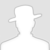\
• 0 文章数
• 0 评论数
• 0 关注者